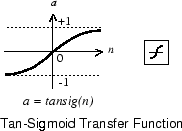# tansig

Hyperbolic tangent sigmoid transfer function

## Graph and Symbol## Syntax

```A = tansig(N,FP) ```

## Description

`tansig` is a neural transfer function. Transfer functions calculate a layer’s output from its net input.

`A = tansig(N,FP)` takes `N` and optional function parameters,

 `N` `S`-by-`Q` matrix of net input (column) vectors `FP` Struct of function parameters (ignored)

and returns `A`, the `S`-by-`Q` matrix of `N`’s elements squashed into `[-1 1]`.

## Examples

Here is the code to create a plot of the `tansig` transfer function.

```n = -5:0.1:5; a = tansig(n); plot(n,a) ```

Assign this transfer function to layer `i` of a network.

```net.layers{i}.transferFcn = 'tansig'; ```

## Algorithms

```a = tansig(n) = 2/(1+exp(-2*n))-1 ```

This is mathematically equivalent to `tanh(N)`. It differs in that it runs faster than the MATLAB implementation of `tanh`, but the results can have very small numerical differences. This function is a good tradeoff for neural networks, where speed is important and the exact shape of the transfer function is not.

## References

Vogl, T.P., J.K. Mangis, A.K. Rigler, W.T. Zink, and D.L. Alkon, “Accelerating the convergence of the backpropagation method,” Biological Cybernetics, Vol. 59, 1988, pp. 257–263